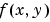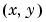Image Processing Toolbox User's Guide# Transforms

The usual mathematical representation of an image is a function of two spatial variables:. The value of the function at a particular locationrepresents the intensity of the image at that point. This is called the spatial domain. The term transform refers to an alternative mathematical representation of an image. For example, the Fourier transform is a representation of an image as a sum of complex exponentials of varying magnitudes, frequencies, and phases. This is called the frequency domain. Transforms are useful for a wide range of purposes, including convolution, enhancement, feature detection, and compression.
This chapter defines several important transforms and shows examples of their application to image processing.
 Fourier Transform Defines the Fourier transform and some of its applications in image processing Discrete Cosine Transform Describes the discrete cosine transform (DCT) of an image and its application, particularly in image compression Radon Transform Describes how the Image Processing Toolbox `radon` function computes projections of an image matrix along specified directions Fan-Beam Projection Data Describes how the Image Processing Toolbox `radon` function computes projections of an image matrix along specified directionsComputing the Frequency Response of a Filter Fourier Transform